Courses

# NEET Previous Year Questions (2014-20): Semiconductor Electronics Notes | EduRev

## Class 12 : NEET Previous Year Questions (2014-20): Semiconductor Electronics Notes | EduRev

The document NEET Previous Year Questions (2014-20): Semiconductor Electronics Notes | EduRev is a part of the Class 12 Course Physics 28 Years Past year papers for NEET/AIPMT Class 12.
All you need of Class 12 at this link: Class 12

Q.1. For transistor action, which of the following statements is correct?    (2020)
A: Both emitter junction as well as the collector junction are forward biased.
B: The base region must be very thin and lightly doped.

C: Base, emitter and collector regions should have same doping concentrations.
D: Base, emitter and collector regions should have same size.

Ans: B
Solution:
For a transistor action, the base junction must be lightly doped so that the base region is very thin.

Q.2. For the logic circuit shown, the truth table is :     (2020)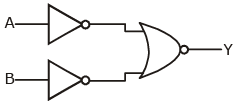A: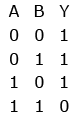B: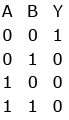C: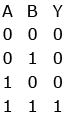D: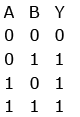Ans: C
Solution: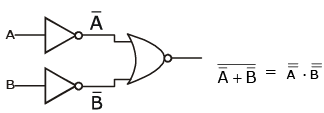= A.B (using de-Morgan’s rule)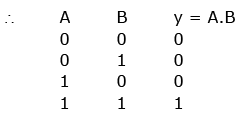Q.3. The increase in the width of the depletion region in a p-n junction diode is due to:     (2020)
A:  both forward bias and reverse bias
B: increase in forward current
C: forward bias only

D: reverse bias only
Ans:
D
Solution:
The increase in the width of the depletion region in a p-n junction diode is due to reverse bias only.

Q.4. A resistance wire connected in the left gap of a metre bridge balances a 10 W resistance in the right gap at a point which divides the bridge wire in the ratio 3 : 2. If the length of the resistance wire is 1.5 m, then the length of 1Ω of the resistance wire is:     (2020)
A: 1.5 × 10-1

B: 1.5 × 10-2 m
C: 1.0 × 10-2 m
D: 1.0 × 10-1 m
Ans:
D
Solution: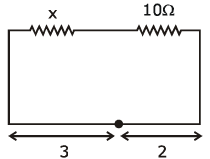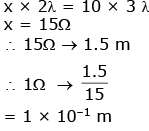Q.5. The correct Boolean operation represented by the circuit diagram drawn is:    (2019)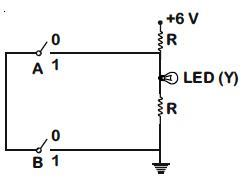A: AND
B: OR
C: NAND
D: NOR
Ans:
C
Solution:

From the given logic circuit LED will glow,
when voltage across LED is high.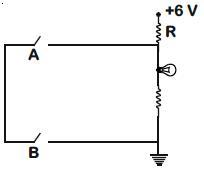Truth Table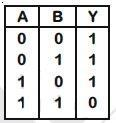This is out put of NAND gate.

Q.6. For a p-type semiconductor, which of the following statements is true ?    (2019)
A: Electrons are the majority carriers and trivalent atoms are the dopants.
B: Holes are the majority carriers and trivalent atoms are the dopants.
C: Holes are the majority carriers and pentavalent atoms are the dopants.
D: Electrons are the majority carriers and pentavalent atoms are the dopants.
Ans:
B
Solution:

In p-type semiconductor, an intrinsic semiconductor is doped with trivalent impurities, that creates deficiencies of valence electrons called holes which are majority charge carriers.

Q.7. In the combination of the following gates the output Y can be written in terms of inputs A and B as :-    (2018)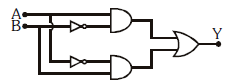A: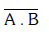B: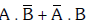C: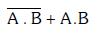D: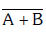Ans: B
Solution: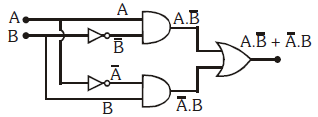Q.8. In a p-n junction diode, change in temperature due to heating :-
A: affects only reverse resistance
B: affects only forward resistance
C: does not affect resistance of p-n junction
D: affects the overall V - I charaterstics of pn junction
Ans:
D
Solution:

Affects the overall V - I characteristic of p-n junction.
On heating, a number of electron - hole pairs increase, so overall resistance of diode will change.
Hence, forward biasing and reversed biasing both are changed.

Q.9. In a common emitter transistor amplifier the audio signal voltage across the collector is 3V. The resistance of collector is 3 kΩ. If current gain is 100 and the base resistance is 2 kΩ, the voltage and power gain of the amplifier is :-    (2017)
A: 15 and 200
B: 150 and 15000
C: 20 and 2000
D: 200 and 1000
Ans:
B
Solution: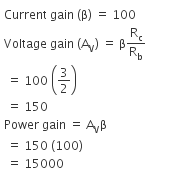Q.10. The given electrical network is equivalent to:    (2017)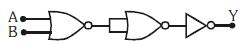A: OR gate
B: NOR gate
C: NOT gate
D: AND gate
Ans:
B
Solution: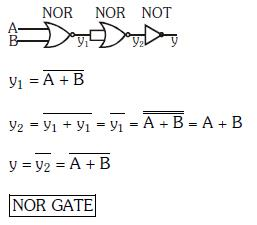Q.11. Which one of the following represents forward bias diode ?     (2017)
A: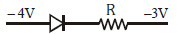B: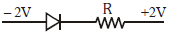C: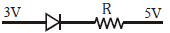D: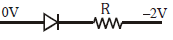Ans:
D
Solution: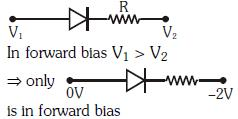Q.12. To get output 1 for the following circuit, the correct choice for the input is :    (2016)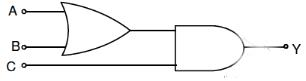A: A = 1, B = 0, C = 1
B: A = 0, B =1, C = 0
C: A = 1, B = 0, C = 0
D: A = 1, B = 1, C = 0
Ans:
A
Solution:

The resultant boolean expression of the above logic circuit is given by,
Y = (A+B).C
Using the inputs given in the options,
If A = 0, B = 0,C = 0, we have
Y = (0+0).0
i.e., Y = 0
If A = 1, B = 1, C = 0, then we have
Y = (1+1).0
i.e., Y = 1.0 = 0
If A = 1, B = 0, C = 1, then
Y = (1+0).1
i.e., Y = 1.1 = 1
If A = 0, B = 1, C = 0, then
Y = (0+1).0
i.e., Y = 1.0
Y = 0
Therefore, output Y = 1 only when inputs A = 1, B = 0 and C = 1.

Q.13. Consider the junction diode as ideal. The value of current flowing through AB is :    (2016)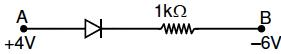A: 10-3 A
B: 0 A
C: 10-2 A
D: 10-1 A
Ans:
C
Solution:
For diode as ideal
I = ΔV/R = 4-(-6)/ 103 = 10-2 A

Q.14. A npn transistor is connected in common emitter configuration in a given amplifier. A load resistance of 800 Ω is connected in the collector circuit and the voltage drop across it is 0.8 V. If the current amplification factor is 0.96 and the input resistance of the circuit is 192Ω , the voltage gain and the power gain of the amplifier will respectively be :    (2016)
A: 4, 3.69
B: 4, 3.84
C: 3.69, 3.84
D: 4, 4
Ans:
B
Solution:

Given,
Resistance across load, RL = 800 ohm
Voltage drop across load, VL = 0.8 V
Input resistance of the circuit, Ri = 192 ohm
Collector current is given by,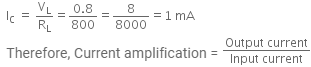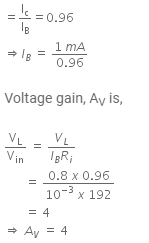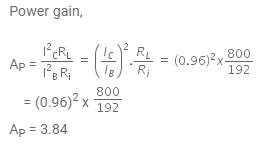Q.15. If in a p-n junction, a square input signal of 10 V is applied, as shown,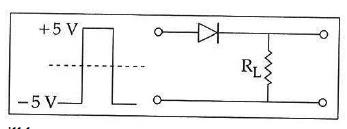then the output across RL will be :     (2015)
A: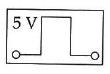B: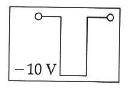C: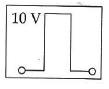D: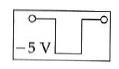Ans:
A
Solution:
As it is forward biased so it takes positive value. Hence, option (A) is correct.

Q.16. Which logic gate is represented by the following combination of logic gates ?    (2015)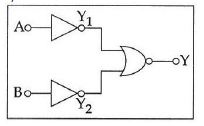A: NOR
B: OR
C: NAND
D: AND
Ans: D
Solution: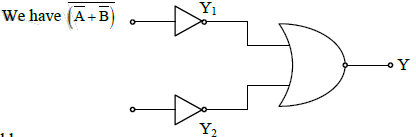Truth table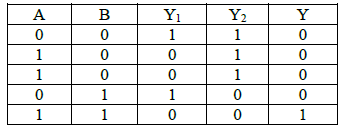This correspond to AND gate

Q.17. The given graph represents V−I characteristic for a semiconductor device.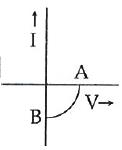Which of the following statement is correct ?    (2014)
A: It is for a photodiode and points A and B represent open circuit voltage and current,
respectively
B: It is for a LED and points A and B represent open circuit voltage and short circuit current, respectively
C: It is V−I characteristic for solar cell where, point A represents open circuit voltage and
point B short circuit current
D: It is for a solar cell and points A and B represent open circuit voltage and current,
respectively
Ans:
C

Q.18. The barrier potential of a p-n junction depends on:    (2014)
(a) type of semi conductor material
(b) amount of doping
(c) temperature
Which one of the following is correct?
A: (b) and (c) only
B: (a),(b) and (c)
C: (a) and (b) only
D: (b) only

Ans: B
Solution:
Barrier potential depends on the material used to make p-n junction diode (whether it is Si or Ge).
It should also depend on the amount of doping due to which the number of majority charge carriers will change. Also, it depends on temperature due to which the number of minority carriers will change.
Barrier potential depends on the material used to make p-n junction diode (whether it is Si or Ge).
It should also depend on the amount of doping due to which the number of majority charge carriers will change. Also, it depends on temperature due to which the number of minority carriers will change.

Offer running on EduRev: Apply code STAYHOME200 to get INR 200 off on our premium plan EduRev Infinity!

14 docs|25 tests

,

,

,

,

,

,

,

,

,

,

,

,

,

,

,

,

,

,

,

,

,

;